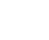# 《表内乘法练习十二》数学二年级上册PPT课件<p>练习十二第四单元表内乘法（一）人教版数学二年级上册完整课件直接使用乘加、乘减运算顺序：不管乘号在前面还是在后面,要先算乘法，再算加减法。如何计算有乘法和加法或者是有乘法和减法的算式？请举例说明。如何计算有乘法和加法或者是有乘法和减法的算式？请举例说明。4&times;5-1=194&times;5+2=①②22①②5&times;3-5=5&times;2=5&times;3+5=5&times;4=5&times;4+5=5&times;5=101020202525计算下面各题。计算下面各题。4&times;2+25=2&times;2-4=4&times;3+12=3&times;3-7=2&times;3+2=4&times;3-2=330242810把下列算式改写成与它结果相同的算式。（1）4+4+4=（）&times;（）（2）5+5+5-6=（）&times;（）-（）（3）5&times;3-5=（）&times;（）（4）2+2+3+2+1+1=（）&times;（）+（）（5）4&times;4+4=（...

《表内乘法练习十二》数学二年级上册PPT课件是由用户上传到202资源网，本次作品主题是PPT课件，素材大小为9.71 MB，素材总共有17页，素材格式为pptx。授权方式为VIP用户下载，成为202资源网VIP用户马上下载此素材。PPT文件完整，下载后可编辑，更多数学课件主题素材点击进入。

• 页数：17页
• 大小：9.71 MB
• 编号：10104
• 类型：VIP模板
• 格式：pptx
• 提示：数字产品不支持退货